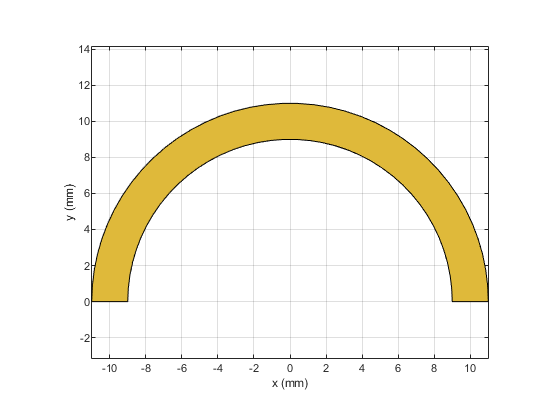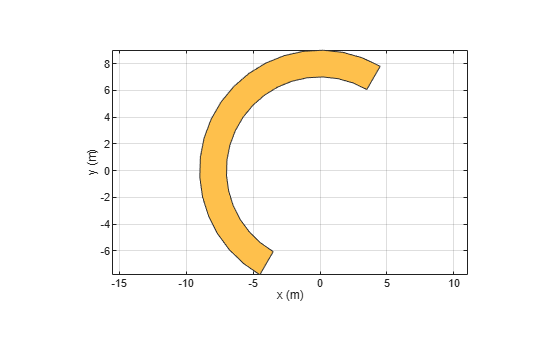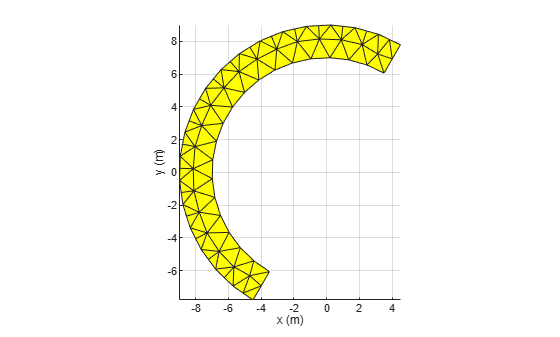# curve

Create curved shape on X-Y plane

Since R2021b

## Description

Use the `curve` object to create a curved shape centered on the X-Y plane.

## Creation

### Syntax

``curvedshape = curve``
``curvedshape = curve(Name=Value)``

### Description

example

````curvedshape = curve` creates a curved shape centered on the X-Y plane.```

example

````curvedshape = curve(Name=Value)` sets Properties using one or more name-value arguments. For example, `curve(ReferencePoint=[1 1])` creates a curved shape at the reference point `[1 1]`. Properties not specified retain their default values.```

## Properties

expand all

Name of the curved shape, specified as a character vector or string scalar.

Example: `curvedshape = curve(Name='curvedshape1')`

Data Types: `char` | `string`

Reference point of the curved shape in Cartesian coordinates, specified as a two-element vector.

Example: `curvedshape = curve(ReferencePoint=[1 1])`

Data Types: `double`

Radius of the curved shape, specified as a positive scalar in meters.

Example: `curvedshape = curve(Radius=0.0300)`

Data Types: `double`

Width of the curved shape, specified as a positive scalar in meters.

Example: `curvedshape = curve(Width=0.0030)`

Data Types: `double`

Start angle and stop angle in degrees, specified as a two-element vector.

Example: `curvedshape = curve(ArcAngle=[90 140)`

Data Types: `double`

## Object Functions

 `add` Boolean unite operation on two RF PCB shapes `and` Shape1 & Shape2 for RF PCB shapes `area` Calculate area of RF PCB shape in square meters `intersect` Boolean intersection operation on two RF PCB shapes `mesh` Change and view mesh properties of metal or dielectric in PCB component `minus` Shape1 - Shape2 for RF PCB shapes `plus` Shape1 + Shape2 for RF PCB shapes `rotate` Rotate RF PCB shape about defined axis `rotateX` Rotate RF PCB shape about x-axis `rotateY` Rotate RF PCB shape about y-axis and angle `rotateZ` Rotate RF PCB shape about z-axis `subtract` Boolean subtraction operation on two RF PCB shapes `scale` Change size of RF PCB shape by fixed amount `show` Display PCB component structure or PCB shape `translate` Move RF PCB shape to new location

## Examples

collapse all

Create a curved shape with default properties.

`curved = curve`
```curved = curve with properties: Name: 'myCurve' ReferencePoint: [0 0] Radius: 0.0100 Width: 0.0020 ArcAngle: [0 180] ```

View the curved shape.

`show(curved)`Create a curved shape with the of 8 m and width of 2 m.

`curved = curve(Radius=8,Width=2);`

Rotate the shape by 60 degrees.

`curved = rotateZ(curved,60)`
```curved = curve with properties: Name: 'myCurve' ReferencePoint: [0 0] Radius: 8 Width: 2 ArcAngle: [0 180] ```
`show(curved)`Mesh the curved shape at a maximum edge length of 1 m.

`mesh(curved,MaxEdgeLength=1)`## Version History

Introduced in R2021b